CBSE (Science) Class 11CBSE
Share

# HC Verma solutions for Class 11 Physics chapter 4 - The Forces

#### HC Verma Concept of Physics Part-1 (2018-2019 Session) by H.C Verma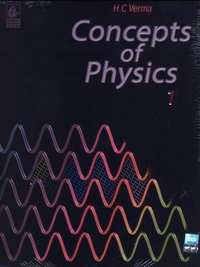## Chapter 4: The Forces

#### HC Verma solutions for Class 11 Physics Chapter 4 Exercise Short Answers [Page 61]

Short Answers | Q 1 | Page 61

A body of mass m is placed on a table. The earth is pulling the body with a force mg. Taking this force to be the action what is the reaction?

Short Answers | Q 2 | Page 61

A boy is sitting on a chair placed on the floor of a room. Write as many action-reaction pairs of forces as you can.

Short Answers | Q 3 | Page 61

A lawyer alleges in court that the police had forced his client to issue a statement of confession. What kind of force is this ?

Short Answers | Q 4 | Page 61

When you hold a pen and write on your notebook, what kind of force is exerted by you on the pen? By the pen on the notebook? By you on the notebook?

Short Answers | Q 5 | Page 61

Is it true that the reaction of a gravitational force is always gravitational, of an electromagnetic force is always electromagnetic and so on?

Short Answers | Q 6 | Page 61

Suppose the magnitude of Nuclear force between two protons varies with the distance between them as shown in figure. Estimate the ratio "Nuclear force/Coulomb force" for
(a) x = 8 fm
(b) x = 4 fm
(c) x = 2 fm
(d) x = 1 fm (1 fm = 10 −15m).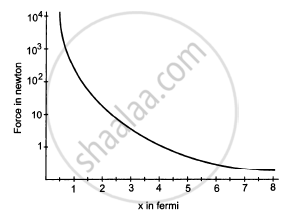Short Answers | Q 7 | Page 61

List all the forces acting on the block B in figure.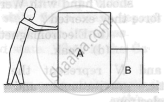Short Answers | Q 8 | Page 61

List all the forces acting on (a) the pulley A, (b) the boy and (c) the block C in figure.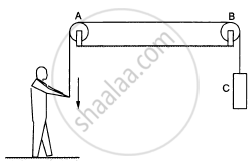Short Answers | Q 9 | Page 61

Figure shows a boy pulling a wagon on a road. List as many forces as you can which are relevant with this figure. Find the pairs of forces connected by Newton's third law of motion.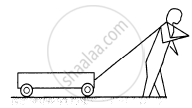Short Answers | Q 10 | Page 61

Figure shows a cart. Complete the table shown below.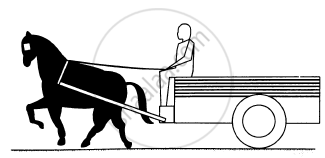Force on Force by Nature of the Force Direction Cart 123: Horse 123: Driver 123:

#### HC Verma solutions for Class 11 Physics Chapter 4 Exercise MCQ [Page 62]

MCQ | Q 1 | Page 62

When Neils Bohr shook hand with Werner Heisenberg, what kind of force they exerted ?

• Gravitational

• Electromagnetic

• Nuclear

• Weak.

MCQ | Q 2 | Page 62

Let E, G and N represent the magnitudes of electromagnetic gravitational and nuclear forces between two electrons at a given separation. Then

• N>E>G

• E>N>G

• G>N>E

• E>G>N

MCQ | Q 3 | Page 62

The sum of all electromagnetic forces between different particles of a system of charged particles is zero

• only if all the particles are positively charged

• only if all the particles are negatively charged

• only if half the particles are positively charged and half are negatively charged

• irrespective of the signs of the charges

MCQ | Q 4 | Page 62

A 60 kg man pushes a 40 kg man by a force of 60 N. The 40 kg man has pushed the other man with a force of

• 40 N

• 0 N

• 60 N

• 20 N

#### HC Verma solutions for Class 11 Physics Chapter 4 Exercise MCQ [Page 62]

MCQ | Q 1 | Page 62

A neutron exerts a force on a proton which is

(a) gravitational
(b) electromagnetic
(c) nuclear
(d) weak

MCQ | Q 2 | Page 62

A proton exerts a force on a proton which is

(a) gravitational
(b) electromagnetic
(c) nuclear
(d) weak

MCQ | Q 3 | Page 62

Mark the correct statements :

(a) The nuclear force between two protons is always greater than the electromagnetic force between them.
(b) The electromagnetic force between two protons is always greater than the gravitational force between them.
(c) The gravitational force between two protons may be greater than the nuclear force between them.
(d) Electromagnetic force between two protons may be greater than the nuclear force acting between them.

MCQ | Q 4 | Page 62

If all matters were made of electrically neutral particles such as neutrons,
(a) there would be no force of friction
(b) there would be no tension in the string
(c) it would not be possible to sit on a chair
(d) the earth could not move around the sun.

MCQ | Q 5 | Page 62

Which of the following systems may be adequately described by classical physics ?

(a) motion of a cricket ball
(b) motion of a dust particle
(c) a hydrogen atom
(d) a neutron changing to a proton.

MCQ | Q 6 | Page 62

The two ends of a spring are displaced along the length of the spring. All displacement have equal magnitudes. In which case or cases the tension or compression in the spring will have a maximum magnitude ?

(a) the right end is displaced towards right and the left end towards left
(b) both ends are displaced towards right
(c) both ends are displaced towards left
(d) the right end is displaced towards left and the left end towards right.

MCQ | Q 7 | Page 62

Action and reaction

(a) act on two different objects
(b) have equal magnitude
(c) have opposite directions
(d) have resultant zero.

#### HC Verma solutions for Class 11 Physics Chapter 4 Exercise [Page 63]

Exercise | Q 1 | Page 63

The gravitational force acting on a particle of 1 g due to a similar particle is equal to 6.67 × 10−17 N. Calculate the separation between the particles.

Exercise | Q 2 | Page 63

Calculate the force with which you attract the earth.

Exercise | Q 3 | Page 63

At what distance should two charges, each equal to 1 C, be placed so that the force between them equals your weight ?

Exercise | Q 4 | Page 63

Two spherical bodies, each of mass 50 kg, are placed at a separation of 20 cm. Equal charges are placed on the bodies and it is found that the force of Coulomb repulsion equals the gravitational attraction in magnitude. Find the magnitude of the charge placed on either body.

Exercise | Q 5 | Page 63

A monkey is sitting on a tree limb. The limb exerts a normal force of 48 N and a frictional force of 20 N. Find the magnitude of the total force exerted by the limb on the monkey.

Exercise | Q 6 | Page 63

A body builder exerts a force of 150 N against a bullworker and compresses it by 20 cm. Calculate the spring constant of the spring in the bullworker.

Exercise | Q 7 | Page 63

A satellite is projected vertically upwards from an earth station. At what height above the earth's surface will the force on the satellite due to the earth be reduced to half its value at the earth station? (Radius of the earth is 6400 km.)

Exercise | Q 8 | Page 63

Two charged particles placed at a separation of 20 cm exert 20 N of Coulomb force on each other. What will be the force of the separation is increased to 25 cm?

Exercise | Q 9 | Page 63

The force with which the earth attracts an object is called the weight of the object. Calculate the weight of the moon from the following data : The universal constant of gravitation G = 6.67 × 11−11 N−m2/kg2, mass of the moon = 7.36 × 1022 kg, mass of the earth = 6 × 1024 kg and the distance between the earth and the moon = 3.8 × 105 km.

Exercise | Q 10 | Page 63

Find the ratio of the magnitude of the electric force to the gravitational force acting between two protons.

Exercise | Q 11 | Page 63

The average separation between the proton and the electron in a hydrogen atom in ground state is 5.3 × 10−11 m. (a) Calculate the Coulomb force between them at this separation. (b) When the atom goes into its first excited state the average separation between the proton and the electron increases to four times its value in the ground state. What is the Coulomb force in this state?

Exercise | Q 12 | Page 63

The geostationary orbit of the earth is at a distance of about 36000 km from the earth's surface. Find the weight of a 120-kg equipment placed in a geostationary satellite. The radius of the earth is 6400 km.

## Chapter 4: The Forces

#### HC Verma Concept of Physics Part-1 (2018-2019 Session) by H.C Verma## HC Verma solutions for Class 11 Physics chapter 4 - The Forces

HC Verma solutions for Class 11 Physics chapter 4 (The Forces) include all questions with solution and detail explanation. This will clear students doubts about any question and improve application skills while preparing for board exams. The detailed, step-by-step solutions will help you understand the concepts better and clear your confusions, if any. Shaalaa.com has the CBSE Concept of Physics Part-1 (2018-2019 Session) by H.C Verma solutions in a manner that help students grasp basic concepts better and faster.

Further, we at Shaalaa.com provide such solutions so that students can prepare for written exams. HC Verma textbook solutions can be a core help for self-study and acts as a perfect self-help guidance for students.

Concepts covered in Class 11 Physics chapter 4 The Forces are Introduction of Work, Energy and Power, Notions of Work and Kinetic Energy: the Work-Energy Theorem, Kinetic Energy, Work Done by a Constant Force and a Variable Force, The Concept of Potential Energy, The Conservation of Mechanical Energy, Potential Energy of a Spring, Various Forms of Energy : the Law of Conservation of Energy, Power, Concept of Collisions, Non - Conservative Forces - Motion in a Vertical Circle, Concept of Work.

Using HC Verma Class 11 solutions The Forces exercise by students are an easy way to prepare for the exams, as they involve solutions arranged chapter-wise also page wise. The questions involved in HC Verma Solutions are important questions that can be asked in the final exam. Maximum students of CBSE Class 11 prefer HC Verma Textbook Solutions to score more in exam.

Get the free view of chapter 4 The Forces Class 11 extra questions for Physics and can use Shaalaa.com to keep it handy for your exam preparation

S